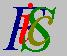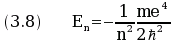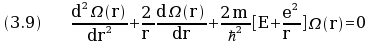Mauro Murzi's pages on Philosophy of Science - Quantum mechanics
Bohr energy levels

# [3. Schrödinger equations.]

## Bohr energy levels.

In Bohr theory of the hydrogen atom, the angular momentum of the electron in its orbit is a multiple of the quantity h/2π, so the energy of the electron is quantized. The values En of the energy of the electron, called the energy levels, are given by the formula.

This formula is derivable from the first Schrödinger equation. Consider an application of the equation (3.7) to the hydrogen atom: The expression for the potential U is U = - e²/r where e is the electron charge and r is the distance from the electron. In polar coordinates the equation (3.7) becamewhere Ω(r) is a function of the distance r. The eigenvalues of the equation (3.9) are the values of E given by equation (3.8). Thus the first Schrödinger equation gives a correct deduction of the energy levels of Bohr theory. Values of E different from series (3.8) generate solutions Ω(r) not acceptable. The figure shows the diagram of four solutions, two acceptable and two not acceptable.